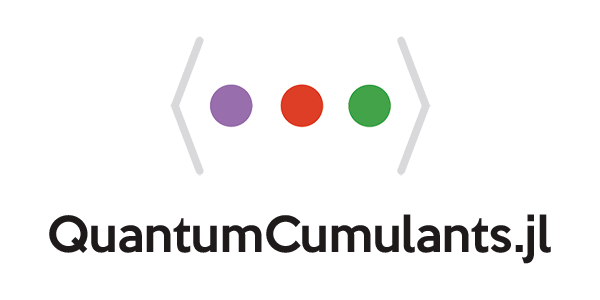QuantumCumulants.jlQuantumCumulants.jl is a package for the symbolic derivation of mean-field equations for quantum mechanical operators in Julia. The equations are derived using fundamental commutation relations of operators. When averaging these equations they can be automatically expanded in terms of cumulants to an arbitrary order (generalized mean-field approximation). This results in a closed set of symbolic differential equations, which can also be solved numerically.

CollectiveSpins.jlCollectiveSpins.jl is a numerical framework written in Julia used to simulate quantum systems consisting of spatially distributed spins coupled via dipole-dipole interaction.

IonSim.jlA lightweight Julia package for simulating the dynamics of a configuration of trapped ions interacting with laser light.